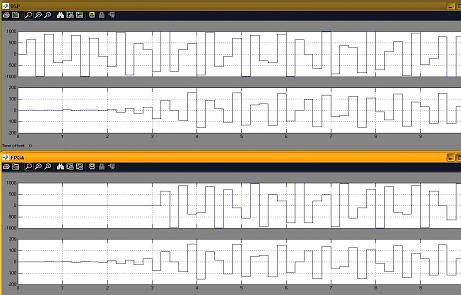# FC202 – Quadrature Conversion

The FC202 is an FPGA core efficiently implementing a quadrature conversion. In order for it to be used within a Diamond DSP/FPGA system, the I/O interfaces are standardized to the model described by 3L Diamond.

The FC202 firmware module is designed to convert a single sampled data channel into its in-phase and quadrature components. This is useful as a pre-cursor for performing a complex Fast Fourier Transform (FFT). This module is designed for flow-through operation from FPGA initialization.

The module was initially developed for correcting all manners of analog data streams sampled by the Beam Forming Reference Design hardware used by a US Navy project but can be used for many other applications too.

### Unit Test Benches

A DSP+FPGA model is implemented using Diamond DSP+FPGA tasks. Samples of a 40MHz sine wave are used as input for the core test benches. With this input FC202 generates the In_Phase and Quadrature components of the real signal. The outputs of the FC202 task are then transferred to the DSP for display on the host. A Simulink model of the core and test-benches were developed in PARS and Simulink. Executing the FC202_mdl.app generates a test.csv file. Graphs for the In-Phase and Quadrature data are generated using excel or Matlab/Simulink.

A GUI model for FC202_simulink.mdl is generated using Simulink, which when run under Matlab will generate the In_Phase and Quadrature components. The scopes display the In_Phase and Quadrature signals. The graphs for the DSP+FPGA and Simulink models should be identical.

### FC202 Quadrature Conversion

In order to interface FC202 to FC108-D module for Polyphase filtering, a conversion from real to quadrature representation is necessary. A firmware module for converting a real signal to an analytic signal of in-phase and quadrature components is wrapped by FC202.

FC202 can also be interfaced to take its input from the FC201 FPGA task, which has been designed for input gain / offset correction.

FC202 is based on linear phase conversion technique, using LogiCore’s Distributed Arithmetic FIR Filter. The basic approach is to approximate the Hilbert Transform using an FIR filter. Typically, when such methods are used, a decimate-by-2 operation can be performed without loss of spectral information. Thus, the data rate of the outputs matches the data rate of the inputs.

The frequency performance of the FC202 is as follows:

 Sample Rate 102.4MHz IF Center 21.4MHz IF Bandwidth 22.5MHz IF Span 10.15MHz  33.65MHz Passband (Gain=1) 5.12MHz  46.08MHz

FC202 integrity test result